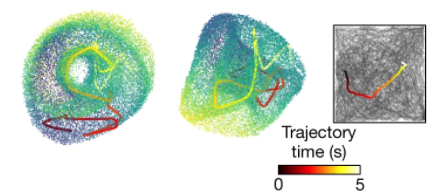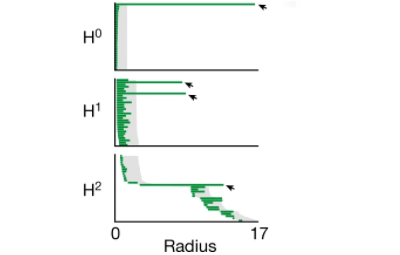# Tony’s Take February 2022

## This month’s topics

### The United Nations and the geometric mean

The Google “geometry” alert for February 7, 2022 pulled up a webpage from the United Nations Development Programme with the title “Why is the geometric mean used for the HDI [Human Development Index] rather than the arithmetic mean?” (Read more about the Human Development Index here, including technical notes.) The arithmetic mean of $n$ numbers $x_1, \dots, x_n$ is just the usual average $\mu = \frac{1}{n}(x_1+\cdots +x_n)$, whereas their geometric mean is $\gamma = \sqrt[n]{x_1 \cdots x_n}$, the $n$th root of their product. The concept of geometric mean goes back at least to Euclid, who used the “mean proportional” (without explicitly defining it) between two lengths $a$ and $b$ to mean the length $c$ such that $a$ is to $c$ as $c$ is to $b$. In modern notation, $a/c=c/b$ so $c^2=ab$ and $c=\sqrt{ab}$, the special case when $n=2$. The geometric mean could also be called the logarithmic mean, since $\log \gamma = \frac{1}{n}(\log x_1 + \cdots + \log x_n)$, which suggests how the geometric mean reduces the impact of larger terms. For example if $x_1=1$ and $x_2 = 100$, then $\mu = 50.5$ whereas $\gamma$ is only 10.

As the UN explains it, they use $\gamma$ in computing the HDI because “Poor performance in any dimension is directly reflected in the geometric mean. In other words, a low achievement in one dimension is not linearly compensated for by a higher achievement in another dimension.” In mathematical terms, for example dividing $x_i$ by $2$ will divide $\gamma$ by $\sqrt[n]{2}$ regardless of the size of $x_i$ compared to the other factors. In human development terms, “a 1 percent decline in the index of, say, life expectancy has the same impact on the HDI as a 1 percent decline in the education or income index.”

### 99 ways to prove your theorem

There are at least 99 styles in which to prove a mathematical statement; they are all illustrated in Philip Ording’s book 99 Variations on a Proof (Princeton, 2019), reviewed by Dan Rockmore (paywall protected) in the New York Review of Books, January 13, 2022. The statement in question is the following:

• If $x^3-6x^2+11x-6=2x-2$, then $x=1$ or $x=4$.

This is not an especially striking specimen of mathematics, but that is the point. Ording is taking us on a mathematical trip following the path of the French literary gymnast Raymond Queneau, whose Exercises de Style, first published in 1947, tells the same unremarkable story (young man with peculiar hat seen arguing on a bus, later spotted getting advice about moving a button on his overcoat) in 99 different styles (telegraphic, exclamatory, botanical, retrograde, …). Rockwell, a mathematics professor at Dartmouth, obviously likes Ording’s book and does his best to bring the NYRB readership around to where they might enjoy it, too. He starts with the assertion “Mathematics is writing,” dragging the subject into their purview (although most other mathematicians would probably not agree with the characterization; he would have been safer with “mathematics almost always involves communication”). And when you have writing you have style: Different mathematicians communicate in very different ways. Ording’s styles, though, like Queneau’s, are not just personal or aesthetic. Rockwell gives us examples, among which:

• #10 Wordless. (See below.)
• #16 Ancient. A proof in Babylonian style, written entirely in Babylonic cuneiform.
• #34 Medieval. Formatted as a medieval manuscript, starts with “Suppose that the intensity of a quality is as the cube of its extension and 9 times that less 6 times its square. It will be demonstrated that when this quality achieves an intensity of 4, its extension is 1 or 4.” (Here the equation is rewritten as $x^3-6x^2+9x=4$.)
• #43 Screenplay. In standard movie-screenplay style, a competition set in 1548 Milan between the algebraic giants of the day, Girolamo Cardano (represented by his protégé Ludovico Ferrari) and Niccolò Tartaglia.

Rockwell quotes extensively from the commentaries that Ording has attached to each proof “outlining the inspiration of the variation and reflecting on the culture of mathematics through the ages.” He adds, “The less mathematically inclined reader might benefit from reading these commentaries first.”#10 Wordless. Rockwell’s review included this wordless proof that $x^3-6x^2+9x-4 = (x-1)^2(x-4)$ and that therefore the polynomial has a root at $x=4$ and a double root at $x=1$. Image by Philip Ording, reproduced with permission.

### “We solved the problem.”

The New York Times on February 1, 2022 included an article by William J. Broad with the headline “A Patron’s Vital Spark Revived a Math Quest.” The quest was the proof of Fermat’s Theorem (that $x^n+y^n=z^n$ has no non-zero integer solutions for $n\geq 3$). As Broad tells us, “according to the usual narrative” the problem was solved by Andrew Wiles toiling in secret for seven years. But in fact the credit should be shared with Mr. James M. Vaughn, Jr., a rich Texan whose money “drew top mathematicians to the puzzle after great minds had given up, succeeded in bringing the moribund field back to life, and may have helped make Dr. Wiles’s breakthrough possible.” James Vaughn himself is less circumspect, and told Broad, “We solved the problem. If we hadn’t put the program together as we did it would still be unsolved.”

Vaughn’s statement is not as far-fetched as it might seem. As Broad explains, Vaughn (who took graduate-level math at the University of Texas) was inspired by Eric Temple Bell’s The Last Problem (1961) and decided to devote part of his considerable inherited wealth to a foundation that would fund research on the problem, starting in 1972. At that time “no mathematician would take his financial aid or even admit to being interested in working on the conundrum.” Vaughn persevered and in 1981 funded a major conference on the topic at MIT, with 76 participants, including the prominent algebraists Kenkichi Iwasawa, Barry Mazur, and Alte Selberg. Andrew Wiles was one of the organizers. Broad calls our attention to the salience of elliptic curve theory among the subjects treated and implies that Vaughn was responsible for this emphasis, which was unpopular among some of the attendees but turned out to be crucial for the final resolution of the problem.

As Broad tells us, one reason that James Vaughn’s role went untold at the time Wiles’s proof was big news is that since 1992 his foundation had switched its support to the arts. Blame it on the IRS and an auditor who was convinced that mathematical research was a boondoggle; Vaughn and his wife were apparently threatened with huge fines and incarceration. As Vaughn put it: “We figured it was too dangerous. You don’t have that kind of trouble if you give to a ballet company.”

### 2D tori in rat neurospace

“Toroidal topology of population activity in grid cells” ran in Nature on January 12, 2022. The authors, an international team led by Richard Gardner and Erik Hermansen (Norwegian University of Science and Technology, Trondheim), are investigating topological models for the firing pattern of grid cells and utilizing topological data analysis techniques. Grid cells reside in the medial entorhinal cortex (MEC), a key component of the neural system that maps an animal’s position within its physical environment. It was known from previous work involving some of the same authors that a grid cell fires, in an individually characteristic hexagonal pattern, depending on the location of the animal. Fig. 1 shows the firing (red dots) of a single grid cell in the MEC of a rat exploring (grey path) a square enclosure. Those authors commented in that earlier paper, “Implausible as the idea might have seemed, cells with regular, periodic place fields are found in the medial entorhinal cortex.”Firing pattern (red dots) of a single grid cell in the MEC of a rat exploring (grey path) a square enclosure. Image from Nature Reviews Neuroscience 7 663–678 (2006), used with permission.

The grid cells are organized in modules. In the new paper, Gardner et al. demonstrate that the periodic place fields of the cells of a single module fit together to map the floor of the enclosure onto a torus. To do this they followed the progress of a rat trained to forage for food in a square enclosure (1.5m on a side); instead of one grid cell as above, they used a set of 149 belonging to the same module. Fig. 2. shows the firing patterns of 36 of those cells. Each of the squares should be compared with Fig. 1. above; it shows how that particular cell is registering the rat’s position in the enclosure. This image and the next two are from the open-source article Nature 602 123-128 (2022). They are used under the Creative Commons license, and have been cropped to fit the format of this column.Fig. 2. Output from 36 of the 149 cells recorded in this experiment. Rat’s path is not shown.

At each instant in time (about four times a second) the outputs from the cells determine a point in 149-dimensional space. The rat’s exploration path thereby generates a cloud of some 30,000 points in what we can call “neurospace.” The team used the latest mathematically motivated, computer-intensive methods to analyze the shape of this cloud.

They first performed principal component analysis, treating the time slots as observations and the cells as variables, to reduce the number of dimensions from 149 to six.

Then they applied UMAP (Uniform Manifold Approximation and Projection) to reduce the number of dimensions to three and allow the cloud to be visualized. UMAP is described by its developers as “a novel manifold learning technique for dimension reduction … constructed from a theoretical framework based in Riemannian geometry and algebraic topology.”Fig. 3. The result of principal component analysis and dimension reduction is this cloud in 3-space (2 views), recognizable as a torus. The rightmost image shows the rat’s exploration path. One 5-second stretch is highlighted; its trace in the cloud is colored to match, illustrating the continuous many-to-one nature of the mapping from the enclosure floor onto the torus.

For an independent check on the topology of that cloud of dots in neurospace, they applied persistent homology theory to the six-dimensional result of principal component analysis. This theory is designed for detecting the topological “shape” of clouds of points, in homological terms: the number of connected components (dimension 0), the number of independent non-bounding closed curves (dimension 1), the number of independent non-bounding closed surfaces (dimension 2), etc. The results are often expressed as a “barcodes” for each dimension, illustrating how structures persist during the course of the analysis. As shown in Fig. 4, persistent homology analysis picks up 1-dimensional zero-th homology, 2-dimensional first homology and 1-dimensional second homology, identifying the cloud as being a 6-dimensional thickening of a 2-dimensional torus.Fig. 4. Persistent homology barcodes for the 6-dimensional cloud of grid-cell firing patterns show one connected component, two independent non-bounding curves and one empty cavity.